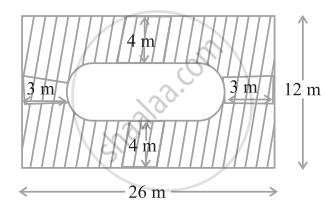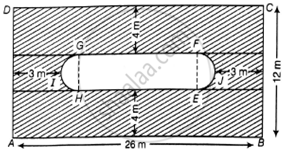# Find the area of the shaded region in figure. - Mathematics

Sum

Find the area of the shaded region in figure.#### SolutionLabel the figure as above.

Area of the shaded region = Area of the rectangle ABCD – (Area of the rect. EFGH + (Area of the semi-circle EFJ + Area of the semi-circle GHI)

Length and breadth of outer rect. ABCD are 26 m and 12 m respectively.

∴ Area of the rect. ABCD = Length × Breadth

= AB × BC

= 26 × 12

= 312 m2

From the figure, length and breadth of inner rect. EFGH are (26 - 5 - 5) m and (12 - 4 - 4) m, i.e. 16 m and 4 m respectively.

∴ Area of the rect. EFGH = Length × Breadth

= EF × FG

= 16 × 4

= 64 m2

Breadth of the inner rectangle = Diameter of the semi-circle EJF = d = 4m

∴ Radius of semi-circle EJF = r = 2 m

Area of the semi-circle EFJ = Area of the semi-circle GHI

= (pir^2)/2

= (4π)/2 = 2π m2

∴ Area of shaded region = 312 – (64 + 2π + 2π)m2

= ( 248 – 4π) m2

Concept: Areas of Combinations of Plane Figures
Is there an error in this question or solution?

#### APPEARS IN

NCERT Mathematics Exemplar Class 10
Chapter 11 Area Related To Circles
Exercise 11.3 | Q 9 | Page 127
Share